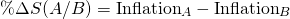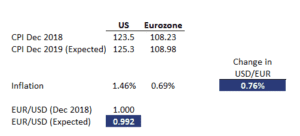# Relative PPP

Relative purchasing power parity (PPP) states that the change in countries’ exchange rate should exactly offset the price effects of inflation differences between the countries. Consider a simple example. Suppose the US has an inflation rate of 2% and the Eurozone has an inflation rate of 0%, then the USD is expected to depreciate by approximately 2% relative to the Euro.

On this page, we discuss the relative purchasing power parity formula, go over a relative PPP example, and finally compare the absolute and relative purchasing power parity. An Excel spreadsheet can be downloaded at the bottom of the page.

## Relative purchasing power parity formula

The relative purchasing power parity definition discussed in the introduction can be expressed as a single formulaThus, the change in the spot price A/B equals the inflation of the price currency (A) minus the inflation of the base currency (B).

## Relative PPP versus absolute PPP

What is the difference between the absolute and relative purchasing power parity? Relative purchasing power parity is based on the argument that, even if absolute PPP (which makes a statement on the level of exchange rates) does not hold, it could still be that changes in exchange rates can be explained by differences in inflation rates between countries over a certain period.

## Relative purchasing power parity example

Next, let’s apply the above formula to calculate the expected change in the exchange rate due to an inflation differential between the two countries. The table below illustrates the necessary calculations using an Excel spreadsheet. As you can see, relative purchasing power parity is really just basic algebra.